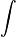# Integration with a rational expression

## Homework Statement

The rate at which people enter an amusement park on a given day is modeled by the function E defined by:

E(t) = 15600/(t² - 24t + 160).

The rate at which people leave the same amusement park on the same day is modeled by the function L defined by:

L(t) = 9890/(t² - 38t +370).

Both E(t) and L(t) are measured in people per hour and time t is measured in hours after midnight. These functions are valid for 9 < t < 23, the hours during which the park is open. At time t = 9, there are no people in the park.

How many people have entered the park by 5:00 pm (t=17)? Round your answer to the nearest whole number.

## The Attempt at a Solution

This problem is actually quite simple. I know I need to take the integral of that function and then solve for C using the information they give me, then plug 17 into the integrated equation.

The only problem is.. integrating that equation is difficult.

I know I can factor out the 15600 in E(t) to get

156001/(t² - 38t +370).

Then...

15600 x ln |(t² - 38t +370)| (because the anti-derivative of 1/x is ln |x|)

That is where I am stuck right now. I know that that isn't the full, complete answer, because if I take the derivative of that equation to check, it doesn't match, because of the chain rule. Am I going about this the wrong way? I don't see any other method, because u-substitution doesn't work, long division won't help, and I don't see how I can break down that equation any further.

I would REALLY like to avoid guess-and-check unless that is the only absolute way I can solve this (which I doubt).

Thanks to any help.. =)

Last edited by a moderator:

cristo
Staff Emeritus
Try completing the square, and then using a trigonometric substitution (the tangent function would be the one to choose here.)

Gib Z
Homework Helper
Ahh the anti derivative of 1/x is ln |x| only when x is a linear function. When its another function, in this case t^2 - 38t etc etc, the antiderivative is different.

Try cristos method, or partial fractions.

Ah, thank you very much for that tip. That worked out much better.

Now, I have a different question. It says that...

H(t) = [PLAIN]http://upload.wikimedia.org/math/a/6/8/a68014a267c9e7ec058affc9f338d7f2.png(from[/URL] [Broken] 9 to t) (E(x) - L(x)) dx for 9 < t < 23. The value of H(17) to the nearest whole number is 3725. Find the value of H'(17), and explain the meaning of H(17) and H'(17) in the context of the amusement park.

Could someone point me in the right direction on how to solve this?

Last edited by a moderator:
H(t) is the number of people in the park at a time t in the park. H'(t) is the rate of people leaving/entering the park in that hour. Solve for H(t).

H'(t) is the rate of people leaving/entering the park in that hour. Solve for H(t).

The derivative of the difference between people leaving and entering.. gives you the rate of people leaving and entering?

That does not make much sense to me.. seeing as it's the difference between the two.

Last edited: